# SOCR EduMaterials AnalysisActivities TwoIndepT

## Two Independent Sample T-Test Example

We will demonstrate Two Independent Wilcoxon Test with a SOCR built-in example. This example is based on the data taken from "Mathematical Statistics and Data Analysis" by John A. Rice, Second Edition, Ducbury Press, 1995. (Page 390)

Here's the steps of the activity:

1. Click on Two Independent Sample T-Test at the left panel's combo box.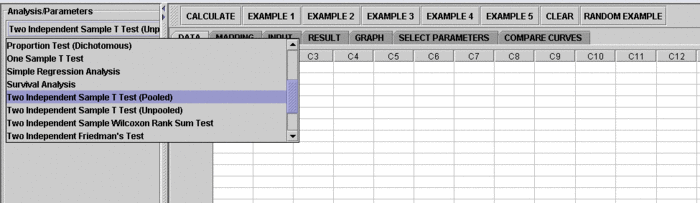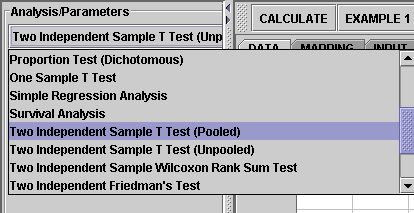On the right panel, first click on EXAMPLE 3 and next click on "Data" to retreive the demonstrated example. This is the example we'll be looking at.Note that the data are difference of B - A.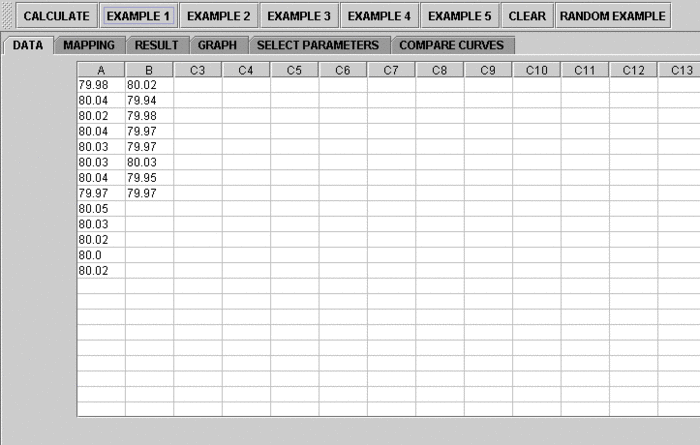2. Click on the "Mapping" button to get to the "Mapping" panel. Click on "ADD" under VARIABLE 1 and to VARIABLE 2 add the variables for Two Independent Sample T-Test. We care about the difference, so which on goes to VARIABLE 1 is arbitrary. You can just add column A to VARIABLE 1 and B to VARIABLE 2. Here, the computer will set difference = Variable 2 - Variable 1. If you like the substraction to go the other way around, simple assign VARIABLE 1 and VARIABLE 2 in a different way.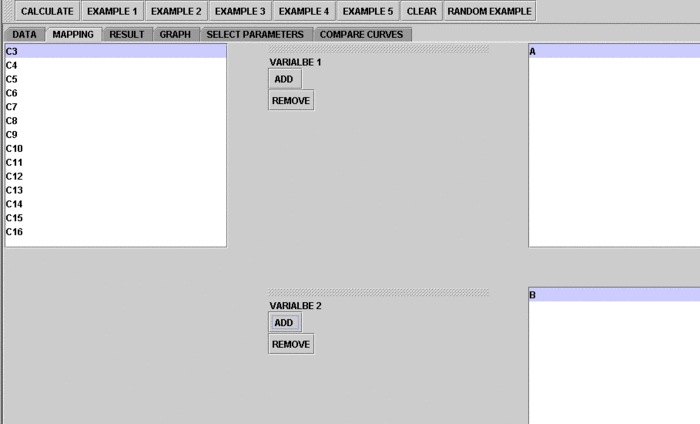3. Click on "Calculate" then "Result" to see the results.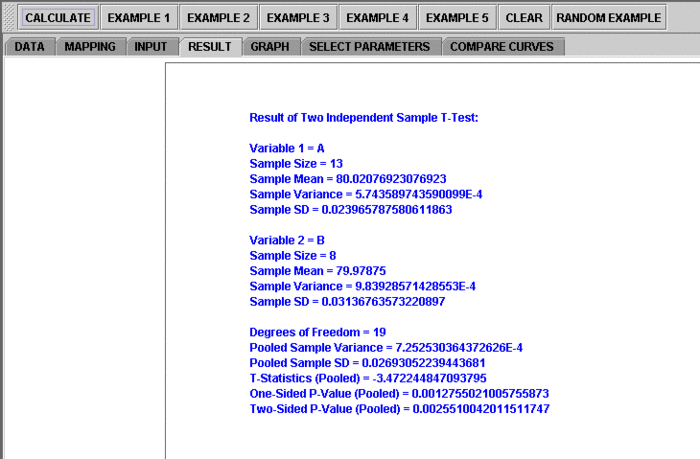Questions for students: By looking at the test results, what do you think about the data? What can you say about the two different shoe materials?